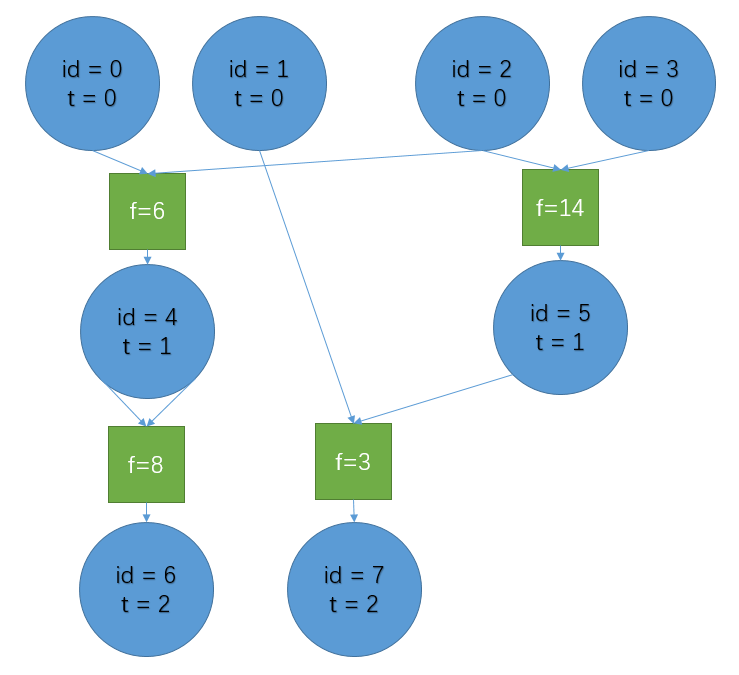# #166. 【清华集训2015】King

$a$$b$$F(a,b,14)$$F(a,b,8)$$F(a,b,6)$
$0$$0$$0$$0$$0$
$0$$1$$1$$0$$1$
$1$$0$$1$$0$$1$
$1$$1$$1$$1$$0$### 任务

• build_computer(task_id, n, M, T)
• task_id: 子任务编号
• n: 输入的$\mathrm{A}$和$\mathrm{B}$的位数
• M: 除了编号小于$2n$的跳蚤之外，最多能使用的跳蚤的数目
• T: 你使用的所有跳蚤的值都要在$\mathrm{T}$小时内被确定

### 子任务

1 $(\mathrm{A} + 1) \mod 2 ^ n$
2 $(\mathrm{A} + \mathrm{B}) \mod 2 ^ n$
3 $(\mathrm{A} \times \mathrm{B}) \mod 2 ^ n$

1 11 $1$ $10000$ $10000$
2 12 $2$ $10000$ $10000$
3 15 $256$ $10000$ $10000$
4 19 $10^5$ $5 \times 10^5$ $50$ 该测试点得分为$\min \left \{ \left \lfloor \frac{5 \times 10^5 - m}{20000} \right \rfloor, 9 \right \}$分
5 110 $10^5$ $1.5 \times 10^6$ $30$ 该测试点得分为$\min \left \{30-t, 10 \right \}$分
6 23 $2$ $10000$ $10000$
7 26 $233$ $10000$ $10000$
8 213$10^5$ $1.2 \times 10^6$ $100$ 该测试点得分为$\min \left \{ \left \lfloor \frac{1.2 \times 10^6-m}{30000} \right \rfloor, 13 \right \}$分
9 214$10^5$ $5 \times 10^6$ $42$该测试点得分为$\min \left \{42-t, 14 \right \}$分
1034 $2$ $10000$ $10000$
1137 $233$ $10^6$ $10^6$
1238 $512$ $3 \times 10^6$ $255$
13317 $1024$ $4 \times 10^6$ $94$ 该测试点得分为$\min \left \{ \left \lfloor\frac{94-t}{2} \right \rfloor, 17 \right \}$分
1431 $1024$ $1920000$ $288$ 第一个通过该测试点的选手将获得一个UOJ抱枕！

### 实现细节

void build_computer(int task_id, int n, int M, int T);

int add_flea(int a, int b, int f);
void set_output(int i, int id);

### 评测方式

1. 第1行：子任务编号
2. 第2行：$n, \mathrm{M}, \mathrm{T}$

build_computer 返回后，如果在 $\mathrm{T}$ 小时后，存在一只跳蚤的值还没被确定，则评测系统输出“Incorrect”， 否则我们将用若干组输入对你造的计算机进行测试， 如果对于所有输入数据，你的输出都正确，评测系统将输出“Correct”，否则输出“Incorrect”。